# 【航迹关联】基于NNDA、PDA、JPDA三种算法实现航迹关联附matlab代码

+关注继续查看

## 2 部分代码

%%%%%%%%%%%%%%%%%%%%%%%%%%%%%%%%%%%%%%%%%%%%%%%%%%%%%%%%%%%%%%%%%%%%%%%%%%%%%%%%%%%%% %generate data of target trajectory %Writed by Liangqun Li  %Date:2006.4.21 %%%%%%%%%%%%%%%%%%%%%%%%%%%%%%%%%%%%%%%%%%%%%%%%%%%%%%%%%%%%%%%%%%%%%%%%%%%%%%%%%%%%% clear all n=50;                                                    %采样次数 T=1;                                                     %T为采样间隔 MC_number=10;                                            %monte carlos run times target_position=[1.5 0.5 1.5  0.1];                      %目标的起始位置和速度                    data_measurement1=zeros(2,n);                            %data_measurement观测值矩阵,data_measurement1实际位置矩阵     Q=[0.0004 0;0 0.0004]; Qdelta=sqrt(Q(1,1)); data_measurement1(:,1,1)=target_position(1); data_measurement1(:,2,1)=target_position(3); for i=2:n         if i~=1             data_measurement1(1,i)=data_measurement1(1,1)+T*(i-1)*target_position(2)+rand(1)*Qdelta;                        data_measurement1(2,i)=data_measurement1(2,1)+T*(i-1)*target_position(4)+rand(1)*Qdelta;   %实际位置 不考虑速度         end end plot(data_measurement1(1,:),data_measurement1(2,:),'-'); axis([0 30 1 7])

## 3 仿真结果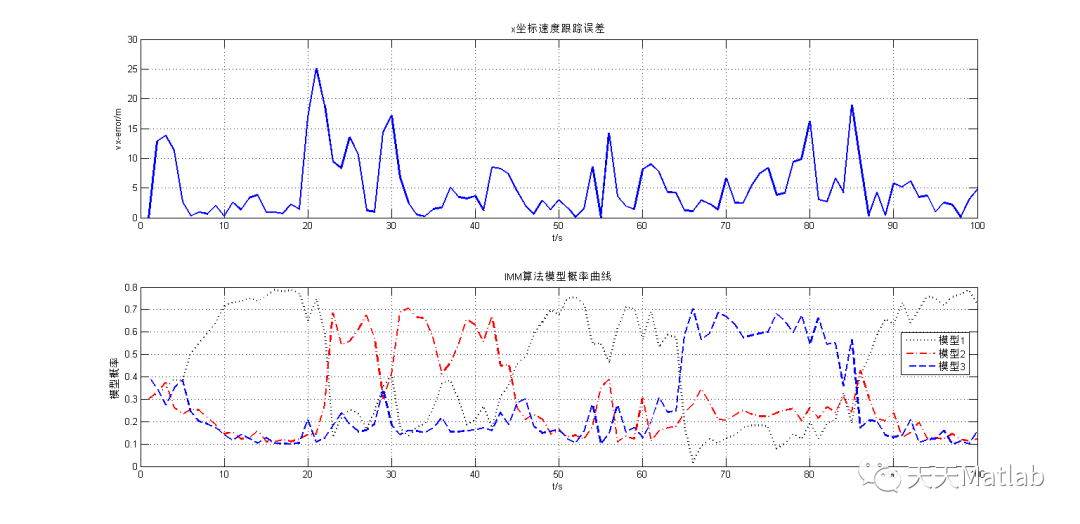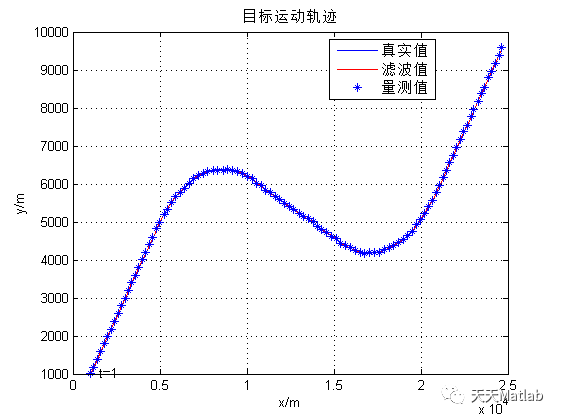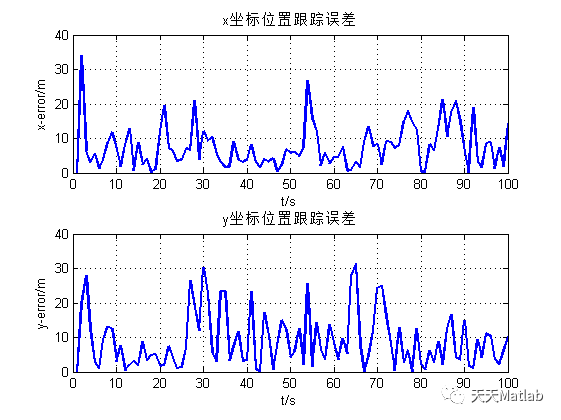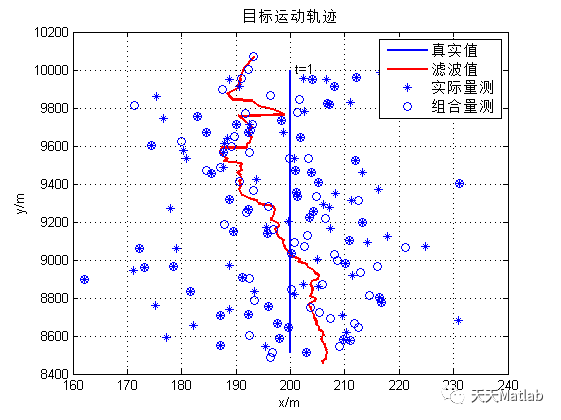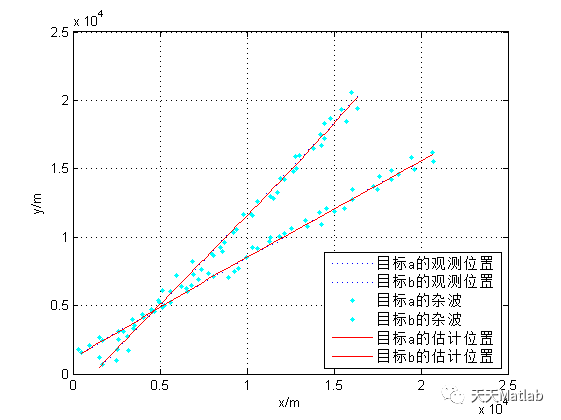## 4 参考文献

宁倩慧, 闫帅, 刘莉,等. 基于JPDA算法的多机动目标航迹跟踪研究[J]. 测试科学与仪器:英文版, 2016.

### 博主简介：擅长智能优化算法、神经网络预测、信号处理、元胞自动机、图像处理、路径规划、无人机等多种领域的Matlab仿真，相关matlab代码问题可私信交流。

#### 部分理论引用网络文献，若有侵权联系博主删除。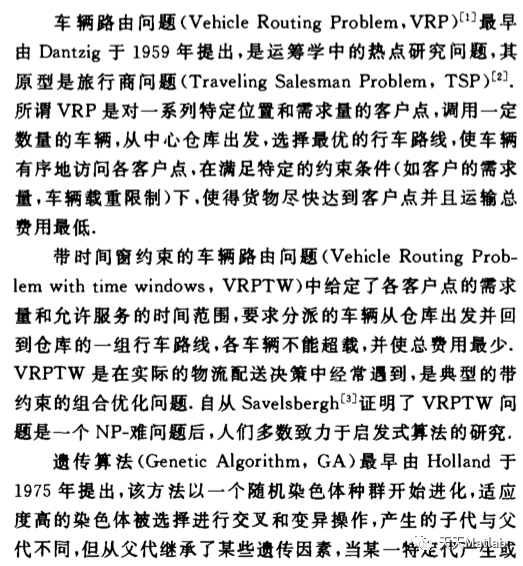【VRP问题】基于遗传算法求解带时间窗车辆路径规划问题（GA-VRPTW）附matlab代码
【VRP问题】基于遗传算法求解带时间窗车辆路径规划问题（GA-VRPTW）附matlab代码
0 0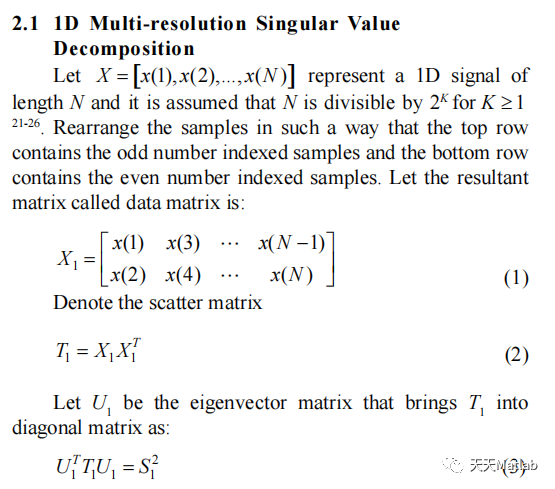0 0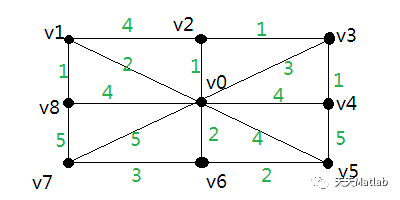0 0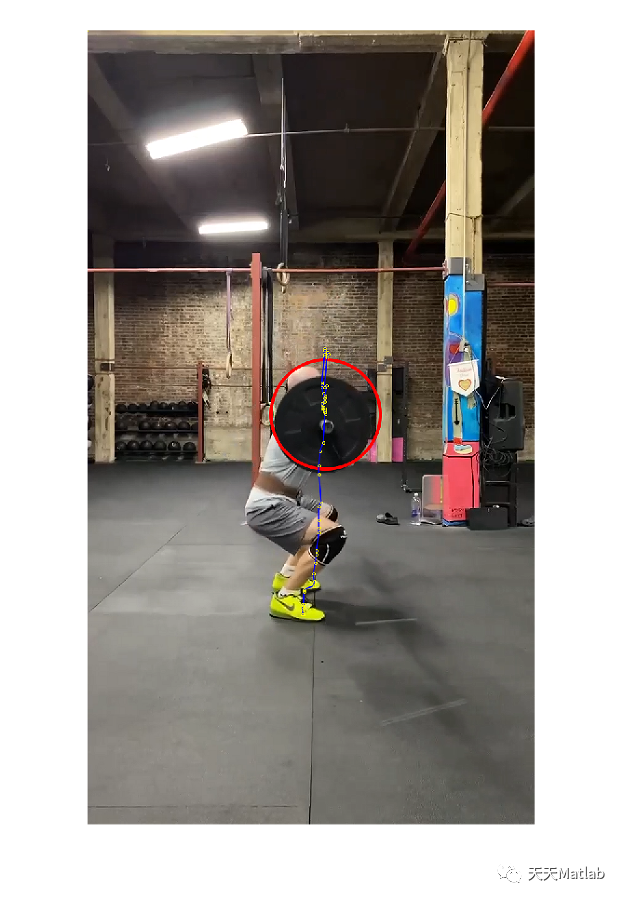【目标检测】基于YOLO神经网络实现人体举重时杠铃的路径和速度跟踪附matlab代码
【目标检测】基于YOLO神经网络实现人体举重时杠铃的路径和速度跟踪附matlab代码
0 0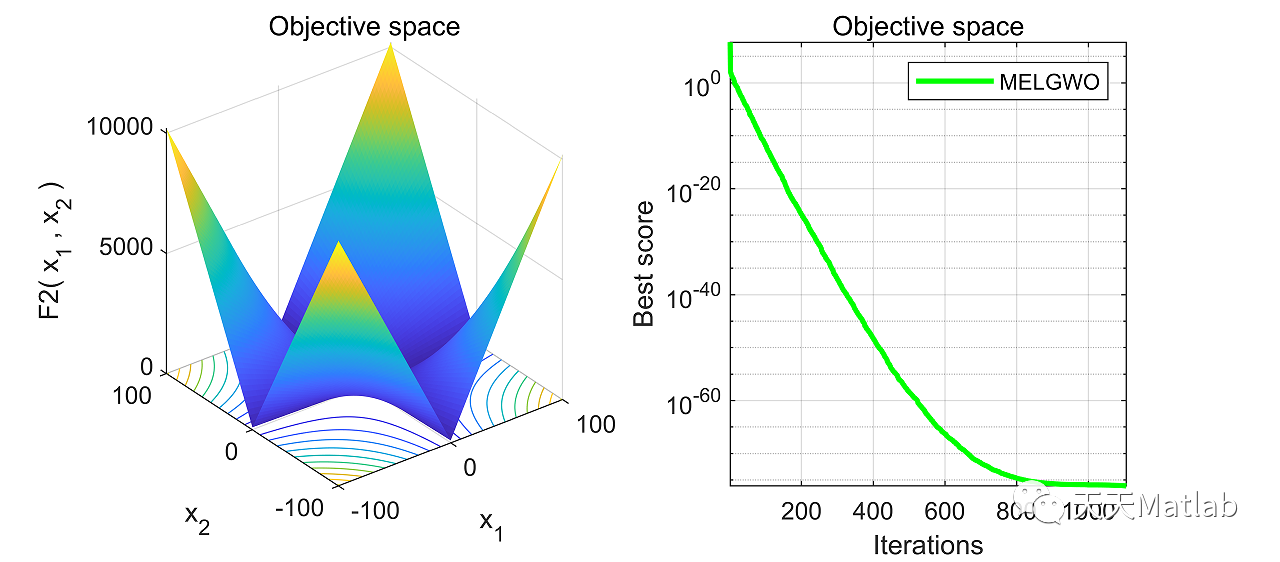【智能优化算法-灰狼算法】基于内存、进化算子和局部搜索的改进灰狼优化器附matlab代码
【智能优化算法-灰狼算法】基于内存、进化算子和局部搜索的改进灰狼优化器附matlab代码
0 0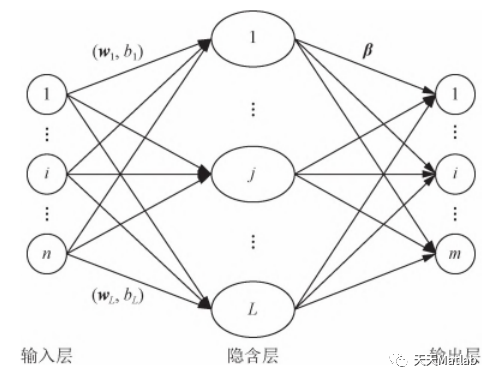【ELM时序预测】基于多尺度集成极限学习机实现寿命预测附matlab代码
【ELM时序预测】基于多尺度集成极限学习机实现寿命预测附matlab代码
0 0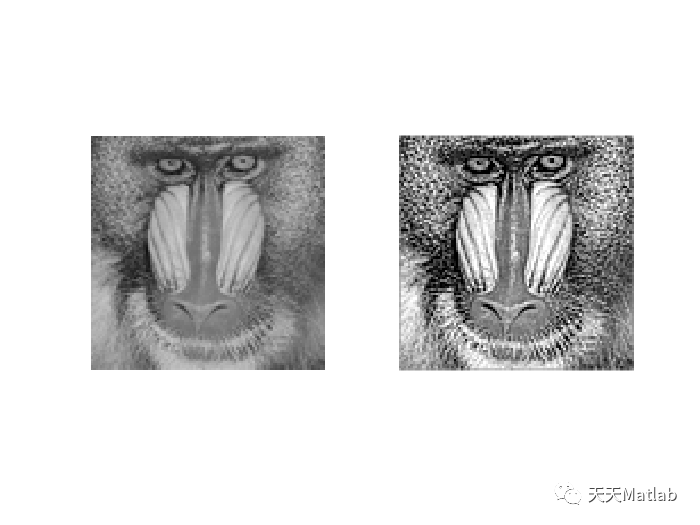【图像增强】基于差分进化和模拟退火实现图像增强附matlab代码
【图像增强】基于差分进化和模拟退火实现图像增强附matlab代码
0 0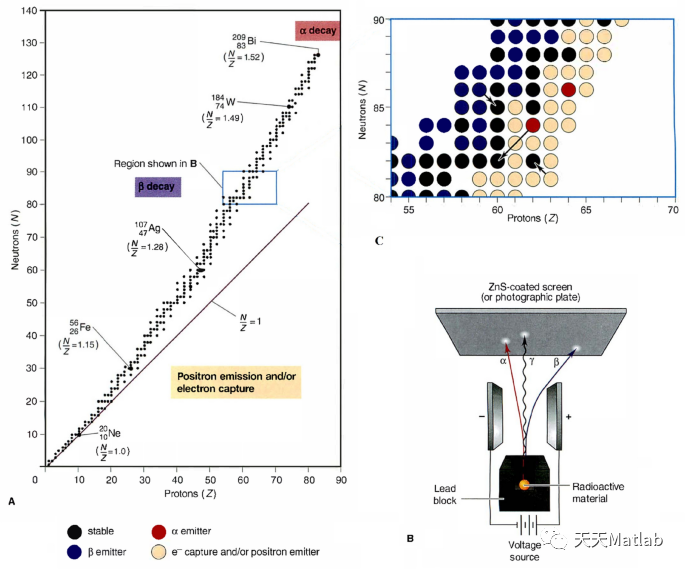0 0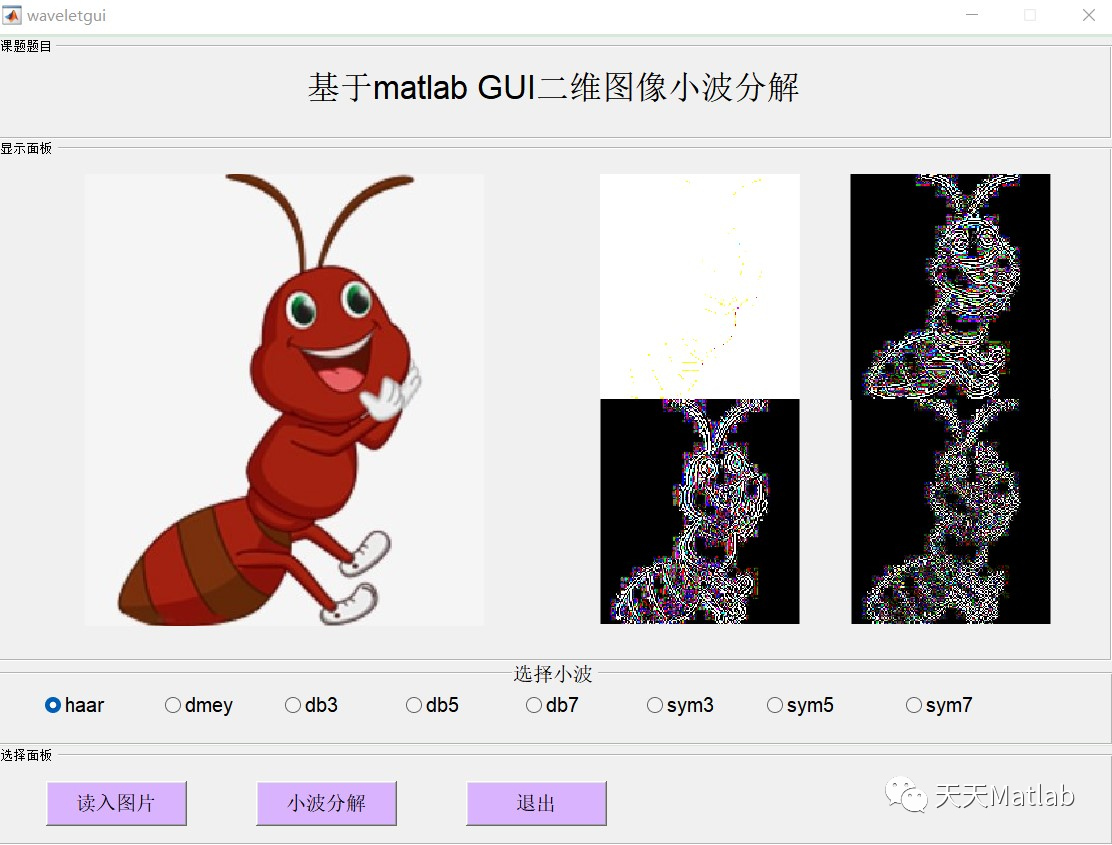【图像分解】基于小波变换实现二维图像分解附matlab代码
【图像分解】基于小波变换实现二维图像分解附matlab代码
0 0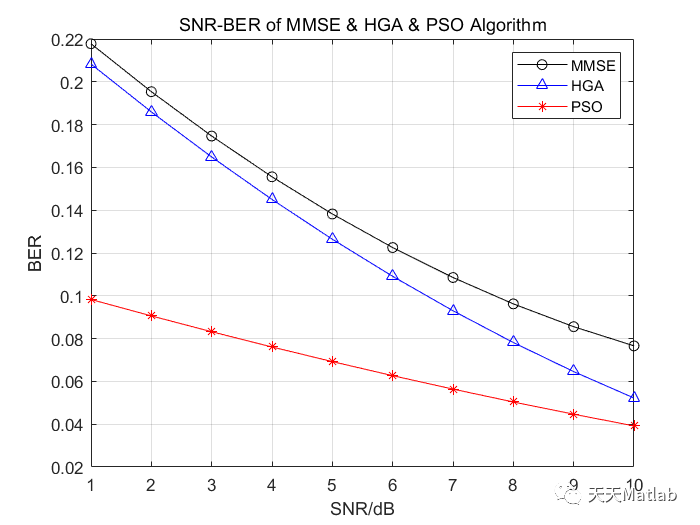【OFDM仿真】基于秩亏情况下遗传算法和粒子群算法优化MIMO-OFDM系统多用户检测附matlab代码
【OFDM仿真】基于秩亏情况下遗传算法和粒子群算法优化MIMO-OFDM系统多用户检测附matlab代码
0 0
Matlab科研工作室